x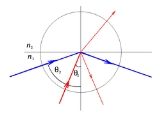Total internal reflectionEncyclopedia
Total internal reflection is an optical phenomenon
Optical phenomenon
An optical phenomenon is any observable event that results from the interaction of light and matter. See also list of optical topics and optics. A mirage is an example of an optical phenomenon....

that happens when a ray of light
Light
Light or visible light is electromagnetic radiation that is visible to the human eye, and is responsible for the sense of sight. Visible light has wavelength in a range from about 380 nanometres to about 740 nm, with a frequency range of about 405 THz to 790 THz...

strikes a medium boundary at an angle larger than a particular critical angle with respect to the normal to the surface. If the refractive index
Refractive index
In optics the refractive index or index of refraction of a substance or medium is a measure of the speed of light in that medium. It is expressed as a ratio of the speed of light in vacuum relative to that in the considered medium....

is lower on the other side of the boundary and the incident angle is greater than the critical angle, no light can pass through and all of the light is reflected
Reflection (physics)
Reflection is the change in direction of a wavefront at an interface between two differentmedia so that the wavefront returns into the medium from which it originated. Common examples include the reflection of light, sound and water waves...

. The critical angle is the angle of incidence
Angle of incidence
Angle of incidence is a measure of deviation of something from "straight on", for example:* in the approach of a ray to a surface, or* the angle at which the wing or horizontal tail of an airplane is installed on the fuselage, measured relative to the axis of the fuselage.-Optics:In geometric...

above which the total internal reflection occurs.

When light crosses a boundary between materials with different refractive indices, the light beam will be partially refracted
Refraction
Refraction is the change in direction of a wave due to a change in its speed. It is essentially a surface phenomenon . The phenomenon is mainly in governance to the law of conservation of energy. The proper explanation would be that due to change of medium, the phase velocity of the wave is changed...

at the boundary surface, and partially reflected. However, if the angle of incidence is greater (i.e. the ray is closer to being parallel to the boundary) than the critical angle – the angle of incidence at which light is refracted such that it travels along the boundary – then the light will stop crossing the boundary altogether and instead be totally reflected back internally. This can only occur where light travels from a medium with a higher [n1=higher refractive index] to one with a lower refractive index [n2=lower refractive index]. For example, it will occur when passing from glass
Glass
Glass is an amorphous solid material. Glasses are typically brittle and optically transparent.The most familiar type of glass, used for centuries in windows and drinking vessels, is soda-lime glass, composed of about 75% silica plus Na2O, CaO, and several minor additives...

to air
Earth's atmosphere
The atmosphere of Earth is a layer of gases surrounding the planet Earth that is retained by Earth's gravity. The atmosphere protects life on Earth by absorbing ultraviolet solar radiation, warming the surface through heat retention , and reducing temperature extremes between day and night...

, but not when passing from air to glass.

## Optical description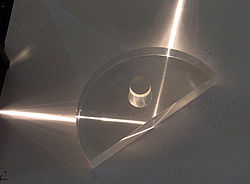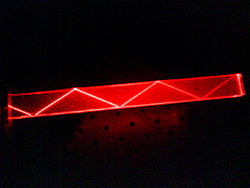Total internal reflections can be demonstrated using a semi-circular glass block. A "ray box" shines a narrow beam of light (a "ray
Ray (optics)
In optics, a ray is an idealized narrow beam of light. Rays are used to model the propagation of light through an optical system, by dividing the real light field up into discrete rays that can be computationally propagated through the system by the techniques of ray tracing. This allows even very...

") onto the glass. The semi-circular shape ensures that a ray pointing towards the centre of the flat face will hit the curved surface at a right angle; this will prevent refraction at the air/glass boundary of the curved surface. At the glass/air boundary of the flat surface, what happens will depend on the angle.
Where θc is the critical angle measurement which is caused by the sun or a light source (measured normal to the surface):
• If θ < θc, the ray will split. Some of the ray will reflect off the boundary, and some will refract as it passes through.
• If θ > θc, the entire ray reflects from the boundary. None passes through. This is called total internal reflection.

This physical property makes optical fiber
Optical fiber
An optical fiber is a flexible, transparent fiber made of a pure glass not much wider than a human hair. It functions as a waveguide, or "light pipe", to transmit light between the two ends of the fiber. The field of applied science and engineering concerned with the design and application of...

s useful and prismatic binoculars
Binoculars
Binoculars, field glasses or binocular telescopes are a pair of identical or mirror-symmetrical telescopes mounted side-by-side and aligned to point accurately in the same direction, allowing the viewer to use both eyes when viewing distant objects...

possible. It is also what gives diamond
Diamond
In mineralogy, diamond is an allotrope of carbon, where the carbon atoms are arranged in a variation of the face-centered cubic crystal structure called a diamond lattice. Diamond is less stable than graphite, but the conversion rate from diamond to graphite is negligible at ambient conditions...

s their distinctive sparkle, as diamond has an extremely high refractive index.

## Critical angle

The critical angle is the angle of incidence above which total internal reflection occurs. The angle of incidence is measured with respect to the normal
Surface normal
A surface normal, or simply normal, to a flat surface is a vector that is perpendicular to that surface. A normal to a non-flat surface at a point P on the surface is a vector perpendicular to the tangent plane to that surface at P. The word "normal" is also used as an adjective: a line normal to a...

at the refractive boundary (see diagram illustrating Snell's law
Snell's law
In optics and physics, Snell's law is a formula used to describe the relationship between the angles of incidence and refraction, when referring to light or other waves passing through a boundary between two different isotropic media, such as water and glass...

).
Consider a light ray passing from glass into air. The light emanating from the interface is bent towards the glass. When the incident angle is increased sufficiently, the transmitted angle (in air) reaches 90 degrees. It is at this point no light is transmitted into air. The critical angle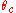is given by Snell's law,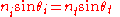.
Rearranging Snell's Law, we get incidence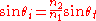.
To find the critical angle, we find the value for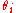when90°. The resulting value ofis equal to the critical angle.

Now, we can solve for, and we get the equation for the critical angle: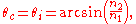If the incident ray is precisely at the critical angle, the refracted ray is tangent
Tangent
In geometry, the tangent line to a plane curve at a given point is the straight line that "just touches" the curve at that point. More precisely, a straight line is said to be a tangent of a curve at a point on the curve if the line passes through the point on the curve and has slope where f...

to the boundary at the point of incidence. If for example, visible light were traveling through acrylic glass (with an index of refraction of 1.50) into air (with an index of refraction of 1.00). The calculation would give the critical angle for light from acrylic into air, which is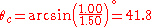.
Light incident on the border with an angle less than 41.8° would be partially transmitted, while light incident on the border at larger angles with respect to normal would be totally internally reflected.

If the fraction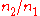is greater than 1, then arcsine is not defined—meaning that total internal reflection does not occur even at very shallow or grazing incident angles.

So the critical angle is only defined whenis less than 1.

## Derivation of evanescent wave

An important side effect of total internal reflection is the propagation of an evanescent wave
Evanescent wave
An evanescent wave is a nearfield standing wave with an intensity that exhibits exponential decay with distance from the boundary at which the wave was formed. Evanescent waves are a general property of wave-equations, and can in principle occur in any context to which a wave-equation applies...

across the boundary surface. Essentially, even though the entire incident wave is reflected back into the originating medium, there is some penetration into the second medium at the boundary. The evanescent wave appears to travel along the boundary between the two materials, leading to the Goos-Hänchen shift.

If a plane wave, confined to the xz plane, is incident on a dielectric
Dielectric
A dielectric is an electrical insulator that can be polarized by an applied electric field. When a dielectric is placed in an electric field, electric charges do not flow through the material, as in a conductor, but only slightly shift from their average equilibrium positions causing dielectric...

with an angle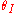and wavevectorthen a transmitted ray will be created with a corresponding angle of transmittance as shown in Fig. 1. The transmitted wavevector is given by: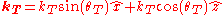If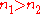, thensince in the relation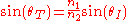obtained from Snell's law
Snell's law
In optics and physics, Snell's law is a formula used to describe the relationship between the angles of incidence and refraction, when referring to light or other waves passing through a boundary between two different isotropic media, such as water and glass...

,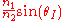is greater than one.

As a result of this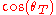becomes complex: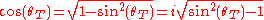The electric field of the transmitted plane wave is given by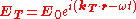and so evaluating this further one obtains:and.

Using the fact that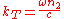and Snell's law, one finally obtains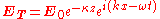whereand.

This wave in the optically less dense medium is known as the evanescent wave. Its characterized by its propagation in the x direction and its exponential attenuation
Attenuation
In physics, attenuation is the gradual loss in intensity of any kind of flux through a medium. For instance, sunlight is attenuated by dark glasses, X-rays are attenuated by lead, and light and sound are attenuated by water.In electrical engineering and telecommunications, attenuation affects the...

in the z direction. Although there is a field in the second medium, it can be shown that no energy flows across the boundary. The component of Poynting vector in the direction normal to the boundary is finite, but its time average vanishes. Whereas the other two components of Poynting vector (here x-component only), and their time averaged values are in general found to be finite.

## Superluminal energy transport

According to a paper published in April 2011 in Optics Communications
Optics Communications
Optics Communications is a peer-reviewed rapid-publication scientific journal published by Elsevier. It reports on all fields of optical science and technology. Articles are available since year 1969. According to the Library of Congress, and CASSI: Vol.1 No...

, the velocity of transfer energy of the transmitted electromagnetic field in the optically thinner medium can exceed the light speed c of vacuum
Faster-than-light
Faster-than-light communications and travel refer to the propagation of information or matter faster than the speed of light....

. However this violates a variety of physical laws.

## Frustrated total internal reflection

Under "ordinary conditions" it is true that the creation of an evanescent wave
Evanescent wave
An evanescent wave is a nearfield standing wave with an intensity that exhibits exponential decay with distance from the boundary at which the wave was formed. Evanescent waves are a general property of wave-equations, and can in principle occur in any context to which a wave-equation applies...

does not affect the conservation of energy, i.e. the evanescent wave transmits zero net energy. However, if a third medium with a higher refractive index
Refractive index
In optics the refractive index or index of refraction of a substance or medium is a measure of the speed of light in that medium. It is expressed as a ratio of the speed of light in vacuum relative to that in the considered medium....

than the low-index second medium is placed within less than several wavelengths distance from the interface between the first medium and the second medium, the evanescent wave will be different from the one under "ordinary conditions" and it will pass energy across the second into the third medium. (See evanescent wave coupling.) This process is called "frustrated" total internal reflection (FTIR) and is very similar to quantum tunneling. The quantum tunneling model is mathematically analogous if one thinks of the electromagnetic field as being the wave function of the photon. The low index medium can be thought of as a potential barrier through which photons can tunnel.

The transmission coefficient for FTIR is highly sensitive to the spacing between the high index media (the function is approximately exponential until the gap is almost closed), so this effect has often been used to modulate optical transmission and reflection with a large dynamic range
Dynamic range
Dynamic range, abbreviated DR or DNR, is the ratio between the largest and smallest possible values of a changeable quantity, such as in sound and light. It is measured as a ratio, or as a base-10 or base-2 logarithmic value.-Dynamic range and human perception:The human senses of sight and...

. An example application of this principle is the multi-touch sensing technology for displays as developed at the New York University’s Media Research Lab.

## Phase shift upon total internal reflection

A lesser-known aspect of total internal reflection is that the reflected light has an angle dependent phase
Phase (waves)
Phase in waves is the fraction of a wave cycle which has elapsed relative to an arbitrary point.-Formula:The phase of an oscillation or wave refers to a sinusoidal function such as the following:...

shift between the reflected and incident light. Mathematically this means that the Fresnel reflection coefficient becomes a complex rather than a real number. This phase shift is polarization dependent and grows as the incidence angle deviates further from the critical angle toward grazing incidence.

The polarization dependent phase shift is long known and was used by Fresnel to design the Fresnel rhomb
Fresnel rhomb
A Fresnel rhomb is a prism-like device designed in 1817 by Augustin-Jean Fresnel for changing the polarization of light waves to be circularly polarized. Though the result is similar to that of using a wave plate, the rhomb does not depend on birefringent properties of the material...

which allows to transform circular polarization
Circular polarization
In electrodynamics, circular polarization of an electromagnetic wave is a polarization in which the electric field of the passing wave does not change strength but only changes direction in a rotary type manner....

to linear polarization
Linear polarization
In electrodynamics, linear polarization or plane polarization of electromagnetic radiation is a confinement of the electric field vector or magnetic field vector to a given plane along the direction of propagation...

and vice versa for a wide range of wavelengths (colors), in contrast to the quarter wave plate
Wave plate
A wave plate or retarder is an optical device that alters the polarization state of a light wave travelling through it.- Operation :A wave plate works by shifting the phase between two perpendicular polarization components of the light wave. A typical wave plate is simply a birefringent crystal...

. The polarization dependent phase shift is also the reason why TE and TM guided modes
Waveguide (optics)
An optical waveguide is a physical structure that guides electromagnetic waves in the optical spectrum. Common types of optical waveguides include optical fiber and rectangular waveguides....

have different dispersion relations.

## Applications

• Optical fiber
Optical fiber
An optical fiber is a flexible, transparent fiber made of a pure glass not much wider than a human hair. It functions as a waveguide, or "light pipe", to transmit light between the two ends of the fiber. The field of applied science and engineering concerned with the design and application of...

s, which are used in endoscopes and telecommunications.
• Rain sensors to control automatic windscreen/windshield wipers.
• Another interesting application of total internal reflection is the spatial filtering of light.
• Prismatic binoculars use the principle of total internal reflections to get a very clear image
• Some multi-touch
Multi-touch
In computing, multi-touch refers to a touch sensing surface's ability to recognize the presence of two or more points of contact with the surface...

screens use frustrated total internal reflection in combination with a camera and appropriate software to pick up multiple targets.
• Gonioscopy
Gonioscopy
Gonioscopy describes the use of a goniolens in conjunction with a slit lamp or operating microscope to gain a view of the iridocorneal angle, or the anatomical angle formed between the eye's cornea and iris...

to view the anatomical angle formed between the eye's cornea
Cornea
The cornea is the transparent front part of the eye that covers the iris, pupil, and anterior chamber. Together with the lens, the cornea refracts light, with the cornea accounting for approximately two-thirds of the eye's total optical power. In humans, the refractive power of the cornea is...

and iris
Iris (anatomy)
The iris is a thin, circular structure in the eye, responsible for controlling the diameter and size of the pupils and thus the amount of light reaching the retina. "Eye color" is the color of the iris, which can be green, blue, or brown. In some cases it can be hazel , grey, violet, or even pink...

.
• Gait analysis
Gait analysis
Gait analysis is the systematic study of animal locomotion, more specific as a study of human motion, using the eye and the brain of observers, augmented by instrumentation for measuring body movements, body mechanics, and the activity of the muscles. Gait analysis is used to assess, plan, and...

instrument, CatWalk, uses frustrated total internal reflection in combination with a high speed camera to capture and analyze footprints of laboratory rodents.
• Fingerprint
Fingerprint
A fingerprint in its narrow sense is an impression left by the friction ridges of a human finger. In a wider use of the term, fingerprints are the traces of an impression from the friction ridges of any part of a human hand. A print from the foot can also leave an impression of friction ridges...

ing devices, which use frustrated total internal reflection in order to record an image of a person's fingerprint without the use of ink.
• Flashlights lenses.

## Examples in everyday life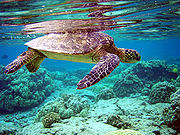Total internal reflection can be observed while swimming, if one opens one's eyes just under the water's surface. If the water is calm, its surface appears mirror-like.

One can demonstrate total internal reflection by filling a sink or bath with water, taking a glass tumbler, and placing it upside-down over the plug hole (with the tumbler completely filled with water). While water remains both in the upturned tumbler and in the sink surrounding it, the plug hole and plug are visible since the angle of refraction between glass and water is not greater than the critical angle. If the drain is opened and the tumbler is kept in position over the hole, the water in the tumbler drains out leaving the glass filled with air, and this then acts as the plug. Viewing this from above, the tumbler now appears mirrored because light reflects off the air/glass interface.

Another very common example of total internal reflection is a critically cut diamond
Diamond
In mineralogy, diamond is an allotrope of carbon, where the carbon atoms are arranged in a variation of the face-centered cubic crystal structure called a diamond lattice. Diamond is less stable than graphite, but the conversion rate from diamond to graphite is negligible at ambient conditions...

. This is what gives it maximum sparkle.

• Evanescent wave
Evanescent wave
An evanescent wave is a nearfield standing wave with an intensity that exhibits exponential decay with distance from the boundary at which the wave was formed. Evanescent waves are a general property of wave-equations, and can in principle occur in any context to which a wave-equation applies...

• Fresnel equations
Fresnel equations
The Fresnel equations , deduced by Augustin-Jean Fresnel , describe the behaviour of light when moving between media of differing refractive indices...

• Goos-Hänchen effect
Goos-Hänchen effect
The Goos–Hänchen effect is an optical phenomenon in which linearly polarized light undergoes a small shift, when totally internally reflected. The shift is perpendicular to the direction of propagation, in the plane containing the incident and reflected beams...

• Perfect mirror
Perfect mirror
A perfect mirror is a theoretical mirror that reflects light perfectly, and doesn't transmit or absorb it.Domestic mirrors are not perfect mirrors as they absorb a significant portion of the light which falls on them....

• Refraction
Refraction
Refraction is the change in direction of a wave due to a change in its speed. It is essentially a surface phenomenon . The phenomenon is mainly in governance to the law of conservation of energy. The proper explanation would be that due to change of medium, the phase velocity of the wave is changed...

• Refractive index
Refractive index
In optics the refractive index or index of refraction of a substance or medium is a measure of the speed of light in that medium. It is expressed as a ratio of the speed of light in vacuum relative to that in the considered medium....

• Snell's window
Snell's window
Snell's window is a phenomenon by which an underwater viewer sees everything above the surface through a cone of light of width of about 96 degrees. This phenomenon is caused by refraction of light entering water, and is governed by Snell's Law...

• Total internal reflection fluorescence microscope
Total internal reflection fluorescence microscope
A total internal reflection fluorescence microscope is a type of microscope with which a thin region of a specimen, usually less than 200 nm, can be observed.-Background:...